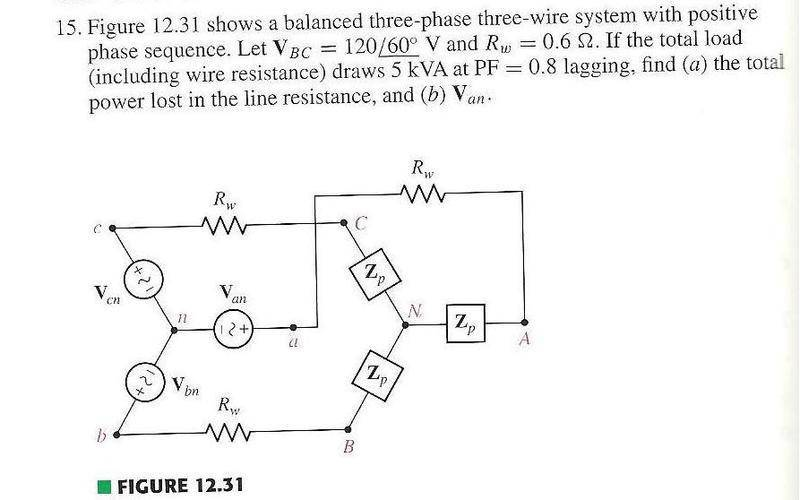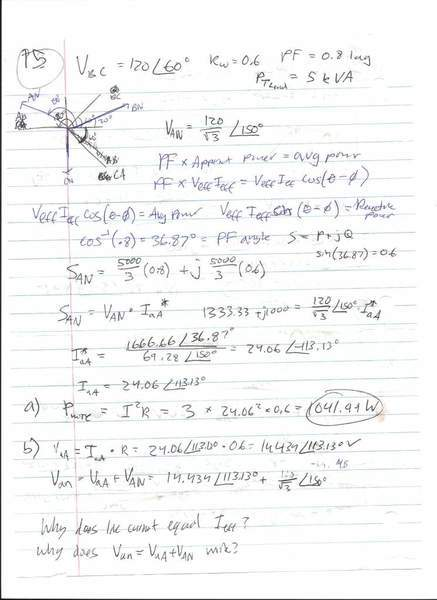# Help with polyphase circuit

• Engineering
Imposta
I'm having some trouble understanding some parts of this questionThis is what I've been doing but I'm not quite sure why it works:I have some similar problems and have been getting the same problems with them. I don't know why the line current equals I(eff) and line voltage equals V(eff). Also why does V(an) = V(aA)+V(AN).

## Answers and Replies

xcvxcvvc
Am I missing something, or is this problem impossible? You're given the line to line voltage at a point after the wire resistance, and the power given includes the load and the wire resistance. After you convert your line to line voltage to a line to neutral voltage, you have a voltage over the load, but you cannot use it to find the line current because of the inclusion of the line resistance in that apparent power given.

For example, you use $$S_{AN}$$ when the given was actually $$S_{AN} + S_{aA}$$

Just think about it: you found the total wire resistance dissipated 1kW of energy, but you are ignoring its effects (which seem to be massive) when you found the line current by assuming it accounted for none of the total load's apparent power.

it would actually be:
$$1333 + j1000 = (V_{AN} + V_{aA}) I^*$$

and we don't know VaA...